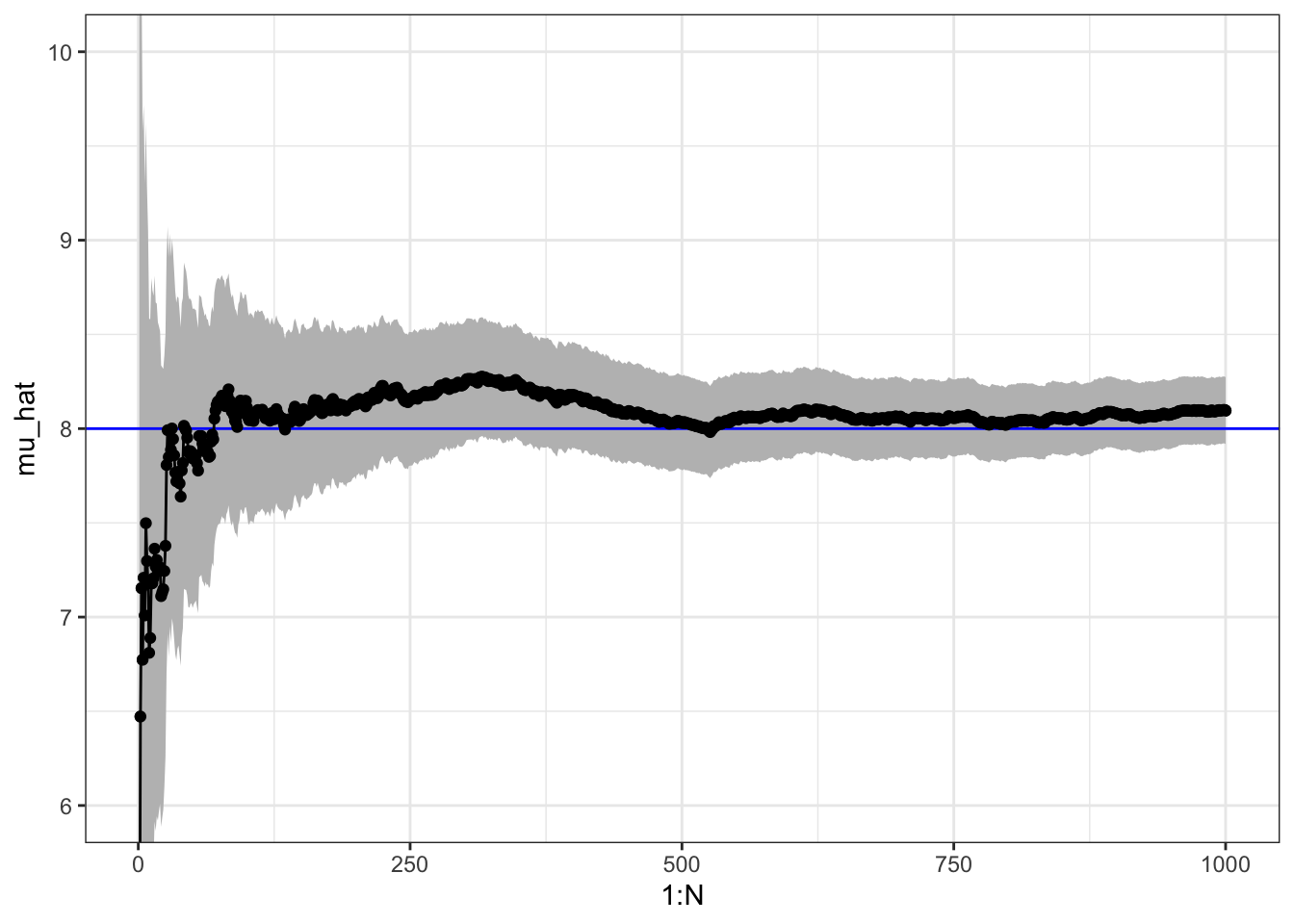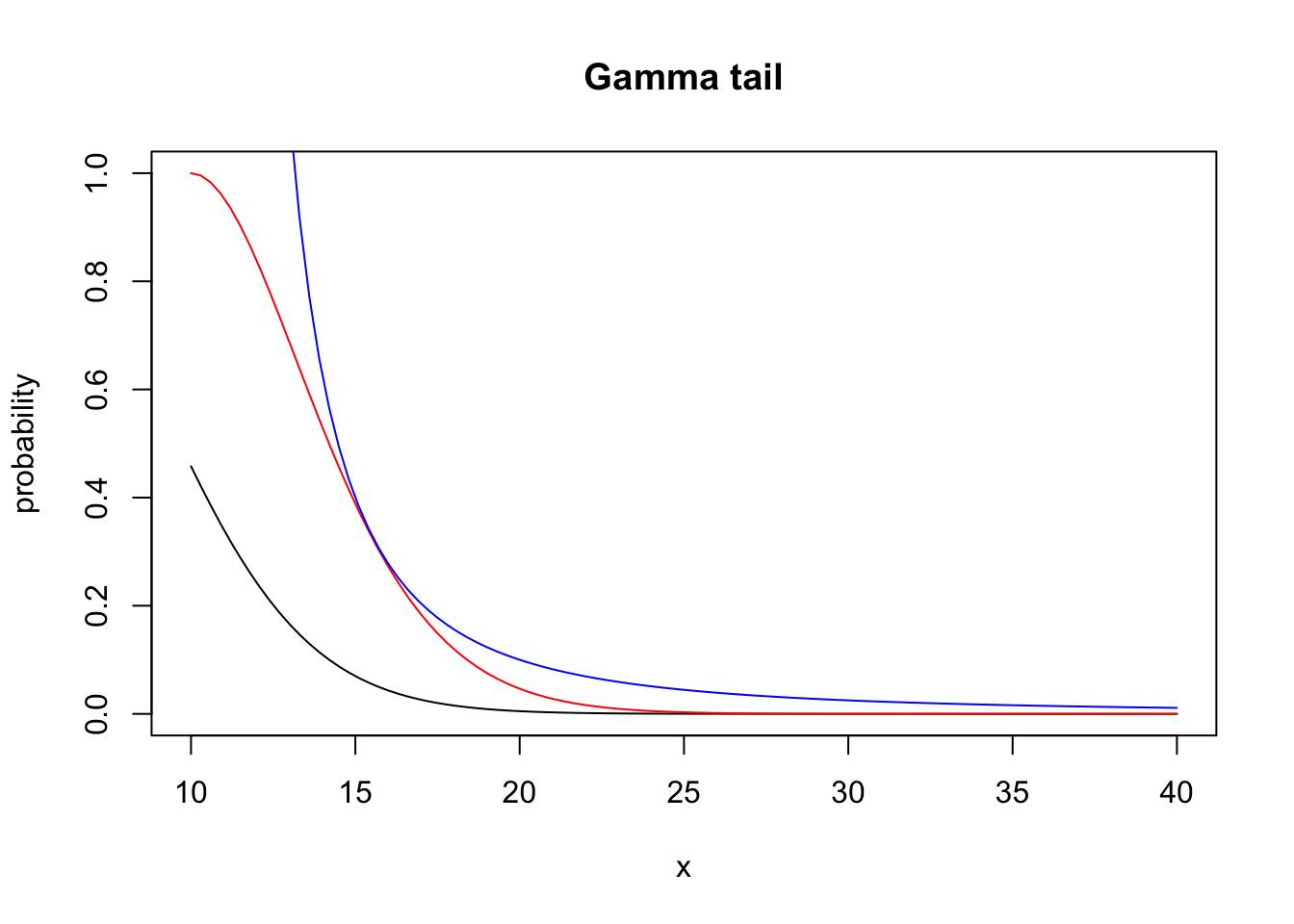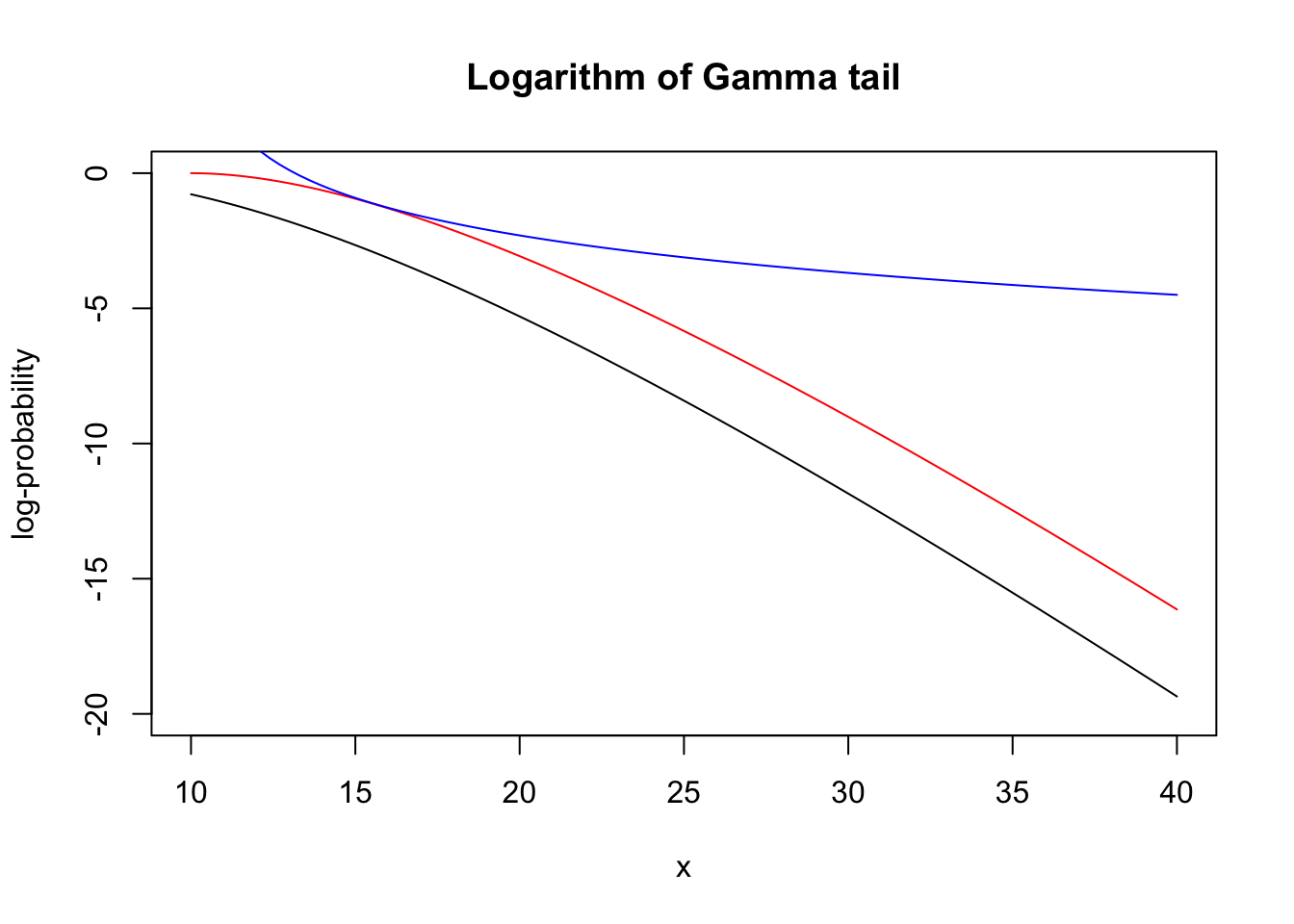## 5.1 Assessment

The error analysis of Monte Carlo integration differs from that of ordinary (deterministic) numerical integration methods. For the latter, error analysis provides bounds on the error of the computable approximation in terms of properties of the function to be integrated. Such bounds provide a guarantee on what the error at most can be. It is generally impossible to provide such a guarantee when using Monte Carlo integration because the computed approximation is by construction (pseudo)random. Thus the error analysis and assessment of the precision of $$\hat{\mu}_{\textrm{MC}}$$ as an approximation of $$\mu$$ will be probabilistic.

There are two main approaches. We can use approximations of the distribution of $$\hat{\mu}_{\textrm{MC}}$$ to assess the precision by computing a confidence interval, say. Or we can provide finite sample upper bounds, known as concentration inequalities, on the probability that the error of $$\hat{\mu}_{\textrm{MC}}$$ is larger than a given $$\varepsilon$$. A concentration inequality can be turned into a confidence interval, if needed, or it can be used directly to answer a question such as: if I want the approximation to have an error smaller than $$\varepsilon = 10^{-3}$$, how large does $$n$$ need to be to guarantee this error bound with probability at least $$99.99$$%?

Confidence intervals are typically computed using the central limit theorem and an estimated value of the asymptotic variance. The most notable practical problem is the estimation of that asymptotic variance, but otherwise the method is straightforward to use. A major deficit of this method is that the central limit theorem does not provide bounds – only approximations of unknown precision for a finite $$n$$. Thus without further analysis, we cannot really be certain that the results from the central limit theorem reflect the accuracy of $$\hat{\mu}_{\textrm{MC}}$$. Concentration inequalities provide actual guarantees, albeit probabilistic. They are, however, typically problem specific and harder to derive, they involve constants that are difficult to compute or estimate, and they tend to be pessimistic in real applications. The focus in this chapter is therefore on using the central limit theorem, but we do emphasize the example in Section 5.2.2 that shows how potentially misleading confidence intervals can be when the convergence is slow.

### 5.1.1 Using the central limit theorem

The CLT gives that $\hat{\mu}_{\textrm{MC}} = \frac{1}{n} \sum_{i=1}^n h(X_i) \overset{\textrm{approx}} \sim \mathcal{N}(\mu, \sigma^2_{\textrm{MC}} / n)$ where $\sigma^2_{\textrm{MC}} = V(h(X_1)) = \int (h(x) - \mu)^2 f(x) \ \mathrm{d}x.$

We can estimate $$\sigma^2_{\textrm{MC}}$$ using the empirical variance $\hat{\sigma}^2_{\textrm{MC}} = \frac{1}{n - 1} \sum_{i=1}^n (h(X_i) - \hat{\mu}_{\textrm{MC}})^2,$

then the variance of $$\hat{\mu}_{\textrm{MC}}$$ is estimated as $$\hat{\sigma}^2_{\textrm{MC}} / n$$ and a standard 95% confidence interval for $$\mu$$ is $\hat{\mu}_{\textrm{MC}} \pm 1.96 \frac{\hat{\sigma}_{\textrm{MC}}}{\sqrt{n}}.$

n <- 1000
x <- rgamma(n, 8) # h(x) = x
mu_hat <- (cumsum(x) / (1:n)) # Cumulative average
sigma_hat <- sd(x)
mu_hat[n]  # Theoretical value 8
##  8.09556
sigma_hat  # Theoretical value sqrt(8) = 2.8284
##  2.861755
qplot(1:n, mu_hat) +
geom_ribbon(
mapping = aes(
ymin = mu_hat - 1.96 * sigma_hat / sqrt(1:n),
ymax = mu_hat + 1.96 * sigma_hat / sqrt(1:n)
), fill = "gray") +
coord_cartesian(ylim = c(6, 10)) +
geom_line() +
geom_point()Figure 5.1: Sample path with confidence band for Monte Carlo integration of the mean of a gamma distributed random variable.

### 5.1.2 Concentration inequalities

If $$X$$ is a real valued random variable with finite second moment, $$\mu = E(X)$$ and $$\sigma^2 = V(X)$$, Chebychev’s inequality holds $P(|X - \mu| > \varepsilon) \leq \frac {\sigma^2}{\varepsilon^2}$ for all $$\varepsilon > 0$$. This inequality implies, for instance, that for the simple Monte Carlo average we have the inequality $P(|\hat{\mu}_{\textrm{MC}} - \mu| > \varepsilon) \leq \frac{\sigma^2_{\textrm{MC}}}{n\varepsilon^2}.$ A common usage of this inequality is for the qualitative statement known as the law of large numbers: for any $$\varepsilon > 0$$ $P(|\hat{\mu}_{\textrm{MC}} - \mu| > \varepsilon) \rightarrow 0$ for $$n \to \infty$$. Or $$\hat{\mu}_{\textrm{MC}}$$ converges in probability towards $$\mu$$ as $$n$$ tends to infinity. However, the inequality actually also provides a quantitative statement about how accurate $$\hat{\mu}_{\textrm{MC}}$$ is as an approximation of $$\mu$$.

Chebyshev’s inequality is useful due to its minimal assumption of a finite second moment. However, it typically doesn’t give a very tight bound on the probability $$P(|X - \mu| > \varepsilon)$$. Much better inequalities can be obtained under stronger assumptions, in particular finite exponential moments.

Assuming that the moment generating function of $$X$$ is finite, $$M(t) = E(e^{tX}) < \infty$$, for some suitable $$t \in \mathbb{R}$$, it follows from Markov’s inequality that $P(X - \mu > \varepsilon) = P(e^{tX} > e^{t(\varepsilon + \mu)}) \leq e^{-t(\varepsilon + \mu)}M(t),$ which can provide a very tight upper bound by minimizing the bound over $$t$$. This requires some knowledge of the moment generating function. We illustrate the usage of this inequality below by considering the gamma distribution where the moment generating function is well known.

### 5.1.3 Exponential tail bound for Gamma distributed variables

If $$X$$ follows a Gamma distribution with shape parameter $$\lambda > 0$$ and $$t <1$$, then $M(t) = \frac{1}{\Gamma(\lambda)} \int_0^{\infty} x^{\lambda - 1} e^{-(1-t) x} \, \mathrm{d} x = \frac{1}{(1-t)^{\lambda}}.$ Whence $P(X-\lambda > \varepsilon) \leq e^{-t(\varepsilon + \lambda)} \frac{1}{(1-t)^{\lambda}}.$ Minimization over $$t$$ of the right hand side gives the minimizer $$t = \varepsilon/(\varepsilon + \lambda)$$ and the upper bound $P(X-\lambda > \varepsilon) \leq e^{-\varepsilon} \left(\frac{\varepsilon + \lambda}{\lambda }\right)^{\lambda}.$ Compare this to the bound $P(|X-\lambda| > \varepsilon) \leq \frac{\lambda}{\varepsilon^2}$ from Chebychev’s inequality.Figure 5.2: Actual tail probabilities (left) for the gamma distribution, computed via the pgamma function, compared it to the tight bound (red) and the weaker bound from Chebychev’s inequality (blue). The differences in the tail are more clearly seen for the log-probabilities (right)